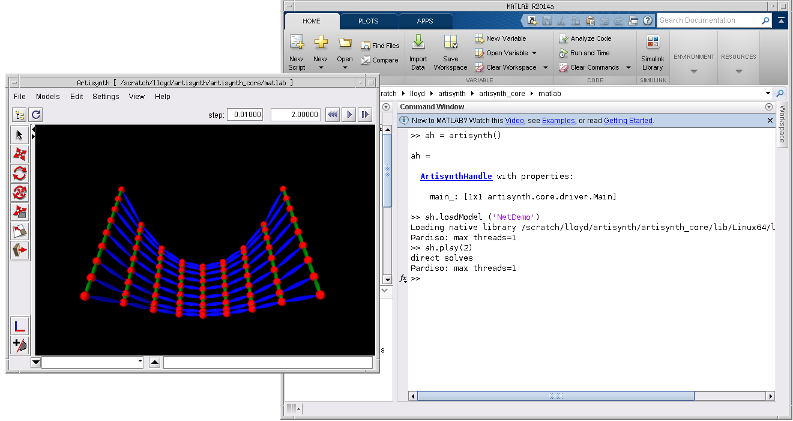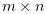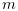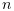# 2 Interfacing to MATLAB

## 2.1 Requirements and configuration

It is probably necessary to use MATLAB 2014 or greater, since only more recent versions of MATLAB use the JOGL 2 Java OpenGL interface which is compatible with ArtiSynth.

In addition, it will also be necessary to set some environment variables. In the following, assume that <ARTISYNTH_HOME> denotes the path to the root folder of the ArtiSynth installation.

1. 1.

If you have not done so already, set the environment variable ARTISYNTH_HOME to <ARTISYNTH_HOME>. This can be done externally to MATLAB (see the Section Environment variables in the ArtiSynth Installation Guide for your system), or it can also be done within a MATLAB startup script using the setenv() command; see the MATLAB documentation regarding startup scripts.

2. 2.

Make sure that the folder <ARTISYNTH_HOME>/matlab is included in the search path for MATLAB functions; consult MATLAB documentation on how to do this.

3. 3.

Ensure that classes required by ArtiSynth are added to the MATLAB Java classpath, as described in Section 2.6.

4. 4.

If you have not done so already, add the ArtiSynth native library folder to the environment variable that specifies the dynamic library search path.

1. (a)

On Windows (64 bit), make sure <ARTISYNTH_HOME>\lib\Windows64 is included in the PATH environment variable.

2. (b)

On Linux (64 bit), make sure <ARTISYNTH_HOME>/lib/Linux64 is included in the LD_LIBRARY_PATH environment variable.

3. (c)

On MacOS, make sure <ARTISYNTH_HOME>/lib/MacOS64 is included in the DYLD_LIBRARY_PATH environment variable.

For more information on setting these variables, see the Section Environment variables in the ArtiSynth Installation Guide appropriate to your system.

5. 5.

Depending on how much memory your ArtiSynth application requires, you may need to adjust the MATLAB Java virtual memory limit (Section 2.5).

6. 6.

As necessary, add the classpath(s) for your Artisynth models to the MATLAB Java classpath, so that they will be visible to Java from within MATLAB. See Section 2.7 for details.

## 2.2 Starting ArtiSynth

Once your environment is set up, you should be able to start MATLAB and then run ArtiSynth by executing the MATLAB command

>> ah = artisynth()

This will start ArtiSynth (Figure 1) and return an ArtisynthHandle object, which can be used to access ArtiSynth structures and data and also provides a number of methods, as described below.Figure 1: ArtiSynth being run from MATLAB.

If desired, the artisynth() command can also accept a variable length set of strings as arguments, corresponding to the options available to the artisynth terminal command. For example,

>> ah = artisynth(’-model’, SpringMeshDemo’)

is equivalent to the terminal command

> artisynth -model SpringMeshDemo

and causes ArtiSynth to look for and load the model named SpringMeshDemo. However, most of what can be achieved using command options can also be achieved by directly accessing ArtiSynth structures or calling handle methods.

Note: at present, if the ArtiSynth GUI is suppressed using the -noGui option, then models must be specified using their fully qualified class names. For SpringMeshDemo, this is artisynth.demos.mech.SpringMeshDemo, and so with the -noGui option the above example would have to be invoked as follows:

   >>> ah = artisynth(’-noGui’, ’-model’, ’artisynth.demos.mech.SpringMeshDemo’)


This is because abbreviated model names are recognized only if ArtiSynth creates the Model menu, which it does not do if the GUI is not invoked. This restriction may change in future versions of ArtiSynth.

To exit ArtiSynth, you can either select File > Quit in the ArtiSynth GUI, or use the quit() method supplied by the handle, as in

>> ah.quit()

After quitting, you can use the artisynth() command to start another ArtiSynth session if desired.

At present, it is not possible to start multiple simultaneous ArtiSynth instances within MATLAB, although that may change in the future.

## 2.3 Querying ArtiSynth structures and models

Through the ArtiSynth handle, it is possible to access most of the Java objects associated with ArtiSynth and its loaded models. Public methods for these objects can be called directly from MATLAB. Java objects can be created by calling their constructors directly, without the need for the keyword new. For example,

>> vec = maspack.matrix.Vector3d (1, 2, 3);

creates a new instance of maspack.matrix.Vector3d and initializes it to (1, 2, 3). As in Java, import statements can be used to allow classes to be specified without using their full package names:

>> import maspack.matrix.*
>>
>> vec = Vector3d (1, 2, 3);

For more details on working with Java objects inside MATLAB, see Call Java Libraries in the MATLAB documentation.

To easily access particular components of a model, the handle method getsel() provides access to to the ArtiSynth selection list. That means you can select items in the ArtiSynth GUI (using either the viewer or navigation panel) and then retrieve these into MATLAB using getsel(). If called with no arguments, getsel() returns the entire selection list as a cell array. If called with an integer argument i, getsel(i) returns the i-th entry in the selection list (where the index i is 1-based).

For example, if two particles are currently selected in ArtiSynth, then getsel() can be used as follows:

>> ah.getsel()       % get entire selection list
ans =
[1x1 artisynth.core.mechmodels.Particle]
[1x1 artisynth.core.mechmodels.Particle]
>> ah.getsel(1)      % get first item on the selection list
artisynth.core.mechmodels.Particle@49752dd4

Once a component has been selected, then one has access to all its public methods. The functions mmat(), amat(), and avec() can be used to map between MATLAB arrays and ArtiSynth Matrix and Vector objects:

mmat(obj)

Creates a MATLAB array from an ArtiSynth Vector or Matrix object. If the Matrix is an instance of SparseMatrix, then mmat() returns a MATLAB sparse matrix. If obj is not a Vector or Matrix, then the method returns the empty matrix.

amat(M)

Creates an ArtiSynth Matrix from a MATLAB array: either a MatrixNd if M is a full matrix, or a SparseMatrixNd if M is sparse.

avec(M)

Creates an ArtiSynth VectorNd from a MATLAB array. At least one of the dimensions of M must be 1.

As a simple example, assume that part refers to an ArtiSynth Particle object. The following code fragment then obtains the particle’s position as a MATLAB array, scales it by 3, and then uses this to reset the position:

>> import maspack.matrix.*
>>
>> pos = mmat(part.getPosition())
pos =
5
0
10
>> pos = 3*pos;
>> part.setPosition (Point3d (avec(pos)));

The particle’s position is returned by the method getPosition(), which returns a Point3d. Since this is an instance of Vector, we use mmat() to turn this into a MATLAB array named pos. After scaling, we turn this back into an ArtiSynth Vector using avec(pos) and reset the particle’s position using the setPosition() method. This method requires a Point3d argument, whereas avec(pos) returns a more general VectorNd object. However, we can create the required Point3d from the VectorNd using the Point3d(Vector) constructor.

As a more complex example, assume that fem refers to an ArtiSynth FemModel3d. The following code fragment then obtains the stiffness matrix for this model and uses MATLAB to find its largest Eigenvalues:

>> K = mmat (fem.getActiveStiffness());
>> eigs (K)
ans =
1.0e+04 *
-8.5443
-8.5443
-6.6442
-6.6442
-5.0636
-4.4762

The current stiffness matrix associated with the active nodes is returned by getActiveStiffness(). Since this is an instance of SparseBlockMatrix, mmat() converts it to a MATLAB sparse matrix, for which we can then find the largest Eigenvalues using eigs().

It is also possible to directly query and set the numeric data associated with ArtiSynth input and output probes. This makes it possible to use MATLAB to plot or process output probe data, or compute and prepare input probe data.

Methods to access probe data are provided by the ArtisynthHandle:

getIprobeData (name);     // get data for the specified input probe
setIprobeData (name, D)   // set data for the specified input probe
getOprobeData (name)      // get data for the specified output probe
setOprobeData (name, D)   // set data for the specified output probe

The probe in question must be a numeric probe, i.e., an instance of NumericProbeBase. name is a string giving either the name or number of the probe. The get() methods return the data as a MATLAB array, while the set() methods receive the data as the MATLAB array D. The data array is, whereis the number of knot points andis the size of the probe’s data vector.

The following example shows the process of obtaining and then changing the numeric data for input probe 0 of the model SpringMeshDemo:

>> D = ah.getIprobeData (’0’)
D =
0  -10.0000         0   20.0000
1.0000         0         0   20.0000
1.9400   10.0000         0    6.8000
3.0000         0         0   10.0000
4.0000  -10.0000         0   20.0000
5.0000         0         0   20.0000
6.0000   10.0000         0   20.0000
7.0000         0         0   10.0000
8.0000  -10.0000         0   20.0000
9.0000         0         0   20.0000
10.0000   10.0000         0   20.0000
11.0000         0         0   10.0000
>> % Now change D by removing all but the first 5 knot points:
>> D = D(1:5,:);
>> ah.setIprobeData (’0’, D);

When setting probe data from MATLAB, the number of columns in the supplied data array must equal the current size of the probe’s data vector.

## 2.4 Scripting commands

The ArtisynthHandle object contains a number of methods that make it possible to load models and control simulation directly from MATLAB, rather than using the ArtiSynth GUI. A full summary of these methods is given Section 4.

In particular, it is possible to load a model and then run or single step a simulation.

To load a model, one may use the method

loadModel (name, args...)

where name is either the fully-qualified classname of the model’s RootModel, or one of the shorter names that appears under the ArtiSynth Models menu, and args... is an optional variable-length list of string arguments that are used to form the args argument of the model’s build() method.

Once loaded, simulation may be controlled using methods such as play(), pause(), step(), or reset(). The following example shows loading a model called RigidBodyDemo and then having it simulate for 2.5 seconds:

>> ah.loadModel (’RigidBodyDemo’);
>> ah.play (2.5);

A particularly powerful feature is the ability to single step execution in a loop, allowing MATLAB to be used to control or inspect the simulation while it is in progress:

>> % single step the simulation for 100 steps
>> for i=1:100
>>    ... adjust inputs if desired ...
>>    ah.step();
>>    ... monitor outputs if desired ...
>> end

This allows MATLAB code to surround each simulation step, assuming a role analogous to the Controller or Monitor objects that can be added to the RootModel. The adjustment of inputs or monitoring of outputs can be accomplished by setting or querying input or output variables of appropriate model components. One way to obtain access to these components is through the find() method discussed above. As a simple example, consider the SimpleMuscleWithController demo describe the ArtiSynth Modeling Guide. The same effect can be achieved by loading SimpleMuscle and then adjusting target position of point p1 directly in MATLAB:

>> import maspack.matrix.Point3d
>> ah.loadModel (’SimpleMuscle’);
>> p1 = ah.find(’models/0/particles/p1’);
>> for i=1:100
>>    ang = ah.getTime()*pi/2;
>>    targ = 0.5*[sin(ang), 0, 1-cos(ang)];
>>    p1.setTargetPosition (Point3d (avec (targ)));
>>    ah.step();
>>    pause (0.01); % slow down simulation if desired
>> end

The call to pause() in the above code simply slows down the simulation so that it appears to run in real time.

## 2.5 Java memory limits

ArtiSynth applications often require a large amount of memory, requiring that the memory limit for the Java virtual machine be set fairly high (perhaps to several gigabytes). By contrast, the default Java memory limit set by MATLAB is often much lower, and so it may be necessary to increase this.

If the memory limit is too low, you may get an out-of-memory error, which generally produces a stack trace on the MATLAB console along with an error message of the form

Exception in thread "AWT-EventQueue-0"
java.lang.OutOfMemoryError: Java heap space

The standard way to increase the MATLAB Java memory limit is from the Preferences menu:

Preferences > MATLAB > General > Java Heap Memory

MATLAB will need to be restarted for any change of settings to take effect.

Unfortunately, at the time of this writing, MATLAB limits the maximum memory size that can be set this way to about 1/4 of the physical memory on the machine, and lower limits have been reported on some systems. If you need more memory than the preferences settings are willing to give you, then you can try creating or editing the java.opts file located in $MATLABROOT/bin/$ARCH, where $MATLABROOT is the MATLAB installation root directory and$ARCH is an architecture-specific directory. Within the java.opts file, you can use the -Xmx option to increase the memory limit. As an example, the following -Xmx settings specify memory limits of 128 Mbytes, 2 Gbytes and 4 Gbytes, respectively:

-Xmx128m
-Xmx2000m
-Xmx4g

More details are given in www.mathworks.com/support/solutions/en/data/1-18I2C.

## 2.6 Setting the classpath for ArtiSynth

Before running ArtiSynth under MATLAB, it is necessary to ensure that the classes used by ArtiSynth are present within MATLAB’s Java classpath. Letting <ARTISYNTH_HOME> denote the path to the top-level directory of the ArtiSynth installation, there are two ways to do this:

1. 1.

Within MATLAB, run the command setArtisynthClasspath with the string associated with <ARTISYNTH_HOME> as an argument. Assuming the environment variable ARTISYNTH_HOME has been set, then the following should work:

  >>> setArtisynthClasspath (getenv (’ARTISYNTH_HOME’))

2. 2.

Create an ASCII text file called javaclasspath.txt in one of the directories in the MATLAB search path, and add to this the path name for <ARTISYNTH_HOME>/classes, as well as the path name for each .jar file in <ARTISYNTH_HOME>/lib. So for example, if <ARTISYNTH_HOME> is /home/joe/artisynth_core, then the entries in javaclasspath.txt would look like this:

  /home/joe/artisynth_core/classes
/home/joe/artisynth_core/lib/quickhull3d.jar
/home/joe/artisynth_core/lib/jython.jar
/home/joe/artisynth_core/lib/jmf.jar
... etc ...


For more details on using javaclasspath.txt, see
www.mathworks.com/help/matlab/matlab_external/static-path.html.

## 2.7 Setting the classpath for models

Usually, ArtiSynth applications involve the use of models or packages defined outside of the ArtiSynth core. In order for these to be visible to Java from inside MATLAB, it is necessary to add their classpaths to MATLAB’s Java classpath. There are three ways to do this:

1. 1.

Add the classpaths to the file EXTCLASSPATH defined in the ArtiSynth root directory. Instructions for doing this are given the section Adding external classes using EXTCLASSPATH of the ArtiSynth Installation Guide.

2. 2.

Add the classpaths within the MATLAB session using the javaaddpath() command. This should be done before the first call to artisynth(), perhaps within a startup script.

3. 3.

Add the classpaths to an ASCII text file called javaclasspath.txt located in one of the directories in the MATLAB search path, as described in Section 2.6.

When adding classpaths for external ArtiSynth models, be sure to add all dependencies. For example, if your model resides under /home/artisynth_projects/classes, but also depends on classes in /home/artisynth_models/classes, then both of these must be added to MATLAB’s Java classpath.

Calling javaaddpath() after the first call to artisynth() may result in some warnings about existing ArtiSynth classes, such as

   Warning: Objects of artisynth/core/driver/Main class exist - not clearing java
> In javaclasspath>doclear at 377


The added classes may also not be visible to ArtiSynth instances created by subsequent invocations of artisynth().

## 2.8 Connecting to an external MATLAB process

In addition to being able to run ArtiSynth from within MATLAB, it is also possible to create a connection between ArtiSynth and an externally running MATLAB program. Data can then be passed back and forth between ArtiSynth and MATLAB. This can be particularly useful if it turns out to be unfeasible to run ArtiSynth directly under MATLAB.

Caveat: The MATLAB connection uses the matlabcontrol interface, by Joshua Kaplan. It relies on the undocumented Java MATLAB Interface, and hence cannot be guaranteed to work with future versions of MATLAB.

An external MATLAB connection can be opened in any of the following ways:

1. 1.

Choosing File > Open MATLAB Connection in the GUI;

2. 2.

Specifying the option -openMatlabConnection on the command line;

3. 3.

Calling the openMatlabConnection() method in the Main class (see Section 2.9).

When a MATLAB connection is requested, the system will first attempt to connect to a MATLAB process running on the user’s machine. If no such process is found, then a new MATLAB process will be started. If a MATLAB connection is opened when ArtiSynth is being run under MATLAB, then the connection will be made to the parent MATLAB process.

Once a connection is open, it is possible to save/load probe data to/from MATLAB. This can be done by selecting the probe and then choosing either Save to MATLAB or Load from MATLAB in the right-click context menu. This will cause the probe data to be saved to (or loaded from) a MATLAB array. The name of the MATLAB array is determined by NumericProbeBase.getMatlabName(), which returns either

1. 1.

The name of the probe, if not null, or

2. 2.

"iprobe<n>" (for input probes) or "oprobe<n>" (for output probes), where <n> is the probe number.

It is also possible to save/load probe data to/from MATLAB using the following functions in the Jython interface (Section 3):

iprobeToMatlab (probeName, matlabName)
iprobeFromMatlab (probeName, matlabName)
iprobeToMatlab (probeName)
iprobeFromMatlab (probeName)
oprobeToMatlab (probeName, matlabName)
oprobeFromMatlab (probeName, matlabName)
oprobeToMatlab (probeName)
oprobeFromMatlab (probeName)

iprobeToMatlab() and iprobeFromMatlab() save/load data for the input probe specified by probeName to/from the MATLAB variable with the name matlabName. probeName is a string giving either the probe’s name or number. If matlabName is absent, then the value of NumericProbeBase.getMatlabName() (described above) is used. The functions oprobeToMatlab() and oprobeFromMatlab() do the same thing for output probes.

## 2.9 Managing an external MATLAB connection from code

The MATLAB connection is associated, internally, with a structure called MatlabInterface, which provides the internal methods for sending data to and from MATLAB. The interface can be obtained and queried from mathods in the Main class:

MatlabInterface openMatlabConnection()

Returns a connection to a MATLAB process. If a connection already exists, that connection is returned. Otherwise, a new connection is established with a MATLAB process running on the user’s machine. If no such process is found, then a new MATLAB process will be started. If ArtiSynth is being run under MATLAB, then the connection will be made to the parent MATLAB process

MatlabInterface getMatlabConnection()

Returns the current MATLAB connection, if any; otherwise, returns null.

boolean hasMatlabConnection()

Returns true if a MATLAB connection currently exists.

boolean closeMatlabConnection()

Closes any current MATLAB connection, returning true if a connection previously existed.

Methods by which a MatlabConnection can send data to and from MATLAB include:

void objectToMatlab (obj, matlabName)

Sets the MATLAB array named matlabName to the values associated with the ArtiSynth object obj. The ArtiSynth object must be either a Matrix, Vector, or double[][]. The assigned MATLAB array is dense, unless the ArtiSynth object is an instance of SparseMatrix, in which the array is sparse.

double[][] arrayFromMatlab (matlabName)

Takes the MATLAB array named by matlabName and returns the corresponding double[][] object, with values assigned in row-major order. If the named MATLAB array does not exist, or is not dense and 2-dimensional, then null is returned.

Matrix matrixFromMatlab (matlabName)

Takes the MATLAB array named by matlabName and returns a corresponding Matrix object. If the named MATLAB array does not exist, or is not 2-dimensional, then null is returned. Otherwise, either a MatrixNd or SparseMatrixNd is returned, depending on whether the array is dense or sparse.

Matrix matrixFromMatlab (matlabName)

Takes the MATLAB array named by matlabName and returns a corresponding VectorNd object. If the named MATLAB array does not exist, or is not 2-dimensional with a size of 1 in at least one dimension, then null is returned.

The above methods are also available in the Jython interface (Section 3) via the functions

objectToMatlab (obj, matlabName)
arrayFromMatlab (matlabName)
matrixFromMatlab (matlabName)
vectorFromMatlab (matlabName)

The following example shows a code fragment in which a MATLAB connection is obtained and then used to transfer data to and from MATLAB:

import artisynth.core.driver.Main;
import artisynth.core.util.MatlabInterface;
...
Main main = Main.getMain();
MatlabInterface mi = main.openMatlabConnection();
// read a matrix MX from MATLAB. Assume we know a-priori that
// MX is dense, so it will be returned as a MatrixNd
MatrixNd MX = (MatrixNd)mi.matrixFromMatlab ("MX");
// send a sparse matrix K to MATLAB and assign it the name KMAT
SparseMatrix K = ...
mi.objectToMatlab (K, "KMAT");